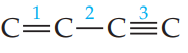×
Get Full Access to Chemistry: The Central Science - 12 Edition - Chapter 8 - Problem 7e
Get Full Access to Chemistry: The Central Science - 12 Edition - Chapter 8 - Problem 7e

×

# The partial Lewis structure that follows is for aISBN: 9780321696724 27

## Solution for problem 7E Chapter 8

Chemistry: The Central Science | 12th Edition

• Textbook Solutions
• 2901 Step-by-step solutions solved by professors and subject experts
• Get 24/7 help from StudySoup virtual teaching assistantsChemistry: The Central Science | 12th Edition

4 5 1 264 Reviews
29
5
Problem 7E

The partial Lewis structure that follows is for a hydrocarbon molecule. In the full Lewis structure, each carbon atom satisfies the octet rule, and there are no unshared electron pairs in the molecule. The carbon–carbon bonds are labeled 1, 2, and 3. (a) Determine where the hydrogen atoms are in the molecule.(b) Rank the carbon–carbon bonds in order of increasing bond length. (c) Rank the carbon–carbon bonds in order of increasing bond enthalpy. [Sections 8.3 and 8.8]Step-by-Step Solution:
Step 1 of 3

q,Co@ + uburla,, L; ge. j*------"t Ch fu tl Dvtn,l,o i \$Ue rr'rriJ*)1. -"----{ Cartoon rkre b.cl0Fovne oQ 6,olo3^co.[ I l"\oks.r\gs, Ir -*tt \iving ofgar4lsvllaga'

Step 2 of 3

Step 3 of 3

## Discover and learn what students are asking

Calculus: Early Transcendental Functions : Surface Integrals
?In Exercises 5 and 6, evaluate $$\int_{S} \int x y d S$$. S: z = 3 - x - y, first octant

Unlock Textbook Solution

Enter your email below to unlock your verified solution to:

The partial Lewis structure that follows is for a